# Lowest-Order Solution

To zeroth order in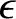, Equation (8.20) yields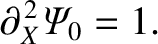(8.54)

Solving this equation subject to the boundary condition (8.40), we obtain(8.55)

Thus, we conclude that, to lowest order in our expansion, the magnetic flux-surfaces in the island region have the constant-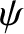structure pictured in Figure 5.7. The island O-points correspond to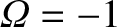and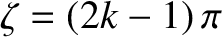(where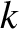is an integer), the X-points correspond to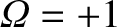and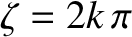, and the magnetic separatrix corresponds to.

To zeroth order in, Equation (8.19) yields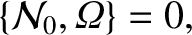(8.56)

where use has been made of Equation (8.55). Given that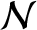is an odd function of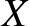, it follows that(8.57)

We conclude that parallel ion acoustic waves, whose dynamics are described by Equation (8.19), smooth out any variations in the lowest-order normalized plasma pressure,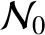, around magnetic flux-surfaces . By symmetry,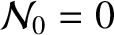inside the magnetic separatrix of the island chain. In other words, the plasma pressure profile (and, by implication, the electron number density, electron temperature, and ion temperature profile—see Section 4.1) is completely flattened inside the separatrix.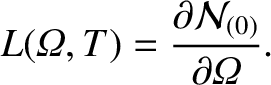(8.58)

It follows that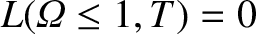. Furthermore, Equations (8.41) and (8.55) imply that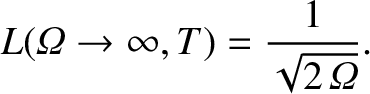(8.59)

To zeroth order in, Equation (8.16) yields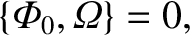(8.60)

where use has been made of Equations (8.55) and (8.57). Given that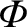is an odd function of, it follows that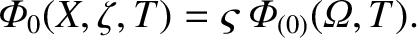(8.61)

We conclude that the lowest-order normalized MHD-fluid stream-function,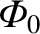, is constant on magnetic flux-surfaces. By symmetry,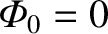inside the magnetic separatrix of the island chain. In other words, the stream-function profile is completely flattened inside the separatrix.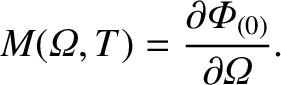(8.62)

It follows that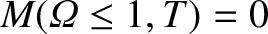. Furthermore, Equations (8.42) and (8.55) imply that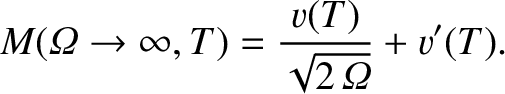(8.63)

To zeroth order in, Equation (8.17) yields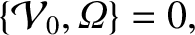(8.64)

where use has been made of Equations (8.55), (8.57), and (8.61). Given that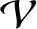is an even function of, we can write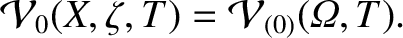(8.65)

In other words, the lowest-order normalized parallel ion velocity,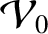, is also constant on magnetic flux-surfaces.

Finally, to zeroth order in, Equation (8.18) yields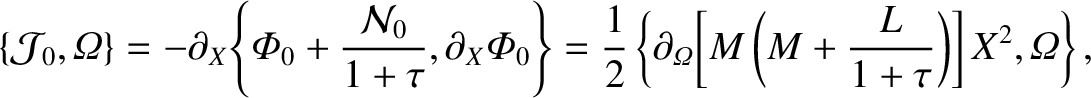(8.66)

where use has been made of Equations (8.55), (8.57), (8.58), (8.61), and (8.62). Moreover,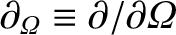.